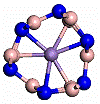## Research Papers in Physics and Astronomy## Stephen Ducharme Publications

#### Date of this Version

December 1987

Published by IEEE. J. of Quantum Electronics 23, 2116 (1987). Permission to use.

#### Abstract

We have measured the low-frequency ("unclamped") electrooptic and piezoelectric coefficients in undoped BaTiO3and SrxBa1-xNb2O6 (x = 0.61) crystals using interferometric techniques. The contribution of the piezoelectric effect to the Pockels measurements is discussed. For an applied ac electric field in the range 0.1-200 V/cm, the electrooptic and piezoelectric effects are linear in the magnitude of the applied field and independent of its frequency in the range 10 Hz-100 kHz. The unclamped electrooptic coefficients of poled BaTiO3 single crystals are r13= 19.5 pm ±1 pm/V and r33 = 97 pm ±7 pm/V, and for strontium barium niobate are r13 = 47 pm ± 5 pm/V and r33 = 235 pm ± 21 pm/V, all measured at a wavelength of 514.5 nm and at T = 23°C. For the barium titanate samples the measured Pockels coefficient rc ≡ r33 - (n1/n3)3 r13 = 79 pm 6 pm/V is in good agreement with the value rc = 76 pm 7 pm/V computed from the above values of r13 and r33, where n1 and n3 are the ordinary and extraordinary indexes of refraction, respectively. The measured piezoelectric coefficient is d23 = +28.7 pm ± 2 pm/V for barium titanate, and is d23 = +24.6 pm ± 2 pm/V for strontium barium niobate. We also measured the photorefractive coupling of two optical beams in the crystals, and we show that the dependence of the coupling strength on beam polarization is in fair agreement with the measured values of the Pockels coefficients.

COinS